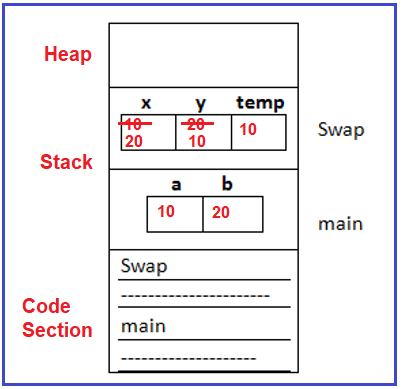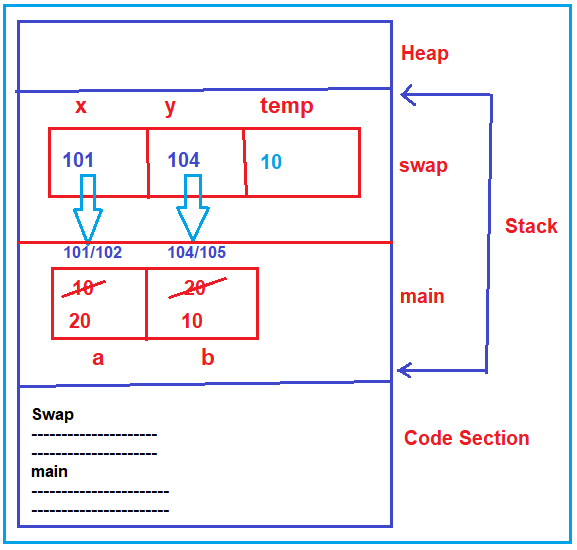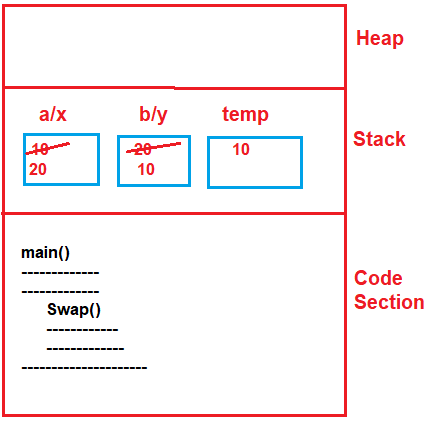# Parameter Passing Methods

## Parameter Passing Methods

In this article, we are going to discuss the parameter passing methods i.e. the 3 parameter passing methods (pass by value, pass by address, and pass by reference). Please read our previous article, where we discussed functions and why should we learn functions. In order to explain all 3 parameter passing methods, we have taken 1 simple example that is the swapping of numbers. So, let us understand all these 3 methods one by one.

##### Pass by value (or) call by value:

In order to understand pass by value or call by value please have a look at the below example. As you can see in the below example, the main function having variable ‘a’ and ‘b’ with the values 10 and 20 respectively. Then the main function calling the swap function. The swap function takes 2 parameters x and y. Then the swap function swapping the numbers x and y with the help of a temporary variable. Then the control comes back to the main function and it will print the values of ‘a’ and ‘b’.

```#include <stdio.h>
void swap (int x, int y)
{
int temp;
temp = x;
x = y;
y = temp;
}

int main ()
{
int a, b;
a = 10;
b = 20;
swap (a, b);
printf ("%d %d", a, b);
}
```
##### How Call by Value work?

In the main function, while calling the swap function we passing two parameters i.e. a and b, and these parameters are called actual parameters. The swap function which takes x and y parameters is called formal parameters.

When the main function called the swap function, the value of x (i.e. 10) and y (i.e. 20) are copied to the x and y variable respectively. Here, the formal parameters are normal variables so takes the values. As the swap function does not return anything so the return type is void. Within the swap function, we have implemented the logic to swap the values of x and y variables using the temp variable.

temp = x; this will store 10 in the temp variable
x = y; this line will store 20 in the x variable
y = temp; here it will store 10 in the y variable.

So, here the formal parameters (x and y) are modified but actual parameters (a and b) remain the same. The swapping is done inside the variables of the swap function only which are not reflected inside actual parameters of the main function.

When the swap function completes its execution, it comes back to the main function from where it is being called. Then inside the main function, it prints the value of a and b and you can see 10 and 20 on the console window. For better understanding please have a look at the below image.So, in the pass-by-value mechanism, any changes are done to formal parameters that will not reflect the actual parameter.

##### When do we need to use Pass by Value?

When we don’t want to modify the actual parameters, then we can use pass by value method. Even though you can also use it when the method returning some value.

So, the swap function should not be done using pass by value. If you want to add two numbers and return the result then in such cases you can use pass by value. But here it is also not suitable for swapping two numbers.

In the call by address mechanism, the addresses of the actual parameters are passed to formal parameters and the formal parameters must be pointers. Any changes that are done with the formal parameters will modify the same with the actual parameters. Now, we need to understand two things, how to write call by value or call by address and how it works?

Note: The point that you need to remember is, call by address uses pointers.

##### Example:

In order to understand the call by address, please have a look at the below example and observe the syntaxes carefully. This is the same swapping example using the call by address. As you can see in the below code, inside the main function while calling the swap function, we are passing the addresses of the actual parameters (i.e. &a and &b), not the values (i.e. 10 and 20). This is the first change i.e. passing the addresses. We know that pointer variables can only hold addresses. So, the second change that we did is we create the formal parameters as pointer variables i.e. (*x and *y).

```#include <stdio.h>
void swap (int *x, int *y)
{
int temp;
temp = *x;
*x = *y;
*y = temp;
}

int main ()
{
int a, b;
a = 10;
b = 20;
swap (&a, &b);
printf ("%d %d", a, b);
}
```

Note: In call by address, the formal parameters must be pointers and here we have to use de-referencing that is *, for accessing the data of actual parameters, and here address will be pass in the case actual parameters.

Now I hope the syntax is clear and let’s proceed and understand the workflow of the call by address.

##### How call by address works?

The main function is having 2 variables ‘a’ and ‘b’ and they are having values 10 and 20 respectively. Next, the swap function is called by the main function and the addresses are passed. In the swap function, the pointers *x and *y will take the addresses. So, now the swap function can access the variables of the main function using pointers.

Note: The point that you need to remember is one function cannot access the variables directly but can access the variables indirectly by using pointers.

Within the swap function, we have the logic to swap the values of the temp variable. Let us see how they work?

temp = *x; this will store 10 in the temp variable. *x will point to the actual value of the address it holds i.e. a variable.
*x = *y; this line will store 20 in the “a” variable. This is because *y points to the b variable and its value are 20 and *x points to the “a” variable.
*y = temp; here it will store 10 in the b variable. This is because *y points to b variable.

So, you can observe that the actual variables are modified. So, when the function swap function ends, the control comes back and when it prints the value of ‘a’ is 20, and the value of ‘b’ is 10 and these are swapped. For better understanding, please have a look at the following diagram.So, call by address is a suitable mechanism for modifying the actual parameters. We will be using this type of code more frequently in our programs. You should be familiar with this one. The call by address is more useful.

##### Call by Reference:

Already we studied in the previous article that the references are supported only in C++. This is not a part of the C language and this is a very useful and powerful mechanism or feature of C++. Reference variables are nothing but an alias to existing variables.

##### How to write the call by reference?

Writing call by references is very easy and very similar to call by value with one small change. In the call by reference, we need to declare the formal parameters using & which will make the formal parameter a reference variable.

For better understanding, please have a look at the below C++ code. As you can see inside the main function, we have two variables ‘a’ and ‘b’ assigned with 1p and 20 respectively. Then the main function calls the swap function by passing these two variables. In the swap function, just before the parameter name or variable name write ‘&’. Now x is a reference to ‘a’ and y is a reference to ‘b’. That means x and y are alternative names for a and b. The reference is used just like a normal variable.

```#include <iostream>
using namespace std;
void swap (int &x, int &y)
{
int temp;
temp = x;
x = y;
y = temp;
}

int main ()
{
int a, b;
a = 10;
b = 20;
swap (a, b);
cout<<a;
cout<<b;
}
```
##### How Call by Reference work?

The program execution starts from the main function. Inside the main function, ‘a’ and ‘b’ are 2 variables and are assigned with 10 and 20 respectively. Then swap function is called by passing ‘a’ and ‘b’ i.e. “a” and “b” are passed to “x” and “y”. Now, x becomes a reference to ‘a’, and y becomes a reference to ‘b’. References are nothing but an alias or another name or a nickname to a variable. References don’t take any memory. References are nothing but another name given to the existing variable.

These two variables are actually belonging to the main function. Main is calling them as and b while the swap function is calling them as x and y. So, the swap is calling with another name. Then within the Swap function, the logic is implemented to swap the numbers. When these formal parameters x and y are manipulated, the actual parameters are modified. This is call by reference

Now one important point we said that one function cannot access the variables of another function directly, it can access indirectly. Then how it is possible to access it directly?

If you see inside the memory, the swap is not a separate function. It has become a part of the main function. And there is only one activation record in the stack frame. For better understanding, please have a look at the following image.As long as the main function code is running, these are ‘a’ and ‘b’, and once the swap function starts these are called x and y and also temp variable is created inside the same old activation record of the main function inside the same stack frame. Once the swap function ends, the temp variable is gone and the values that were 10 and 20 will change to 20 and 10.

So, the swap function is not a separate body of a function. It has become a part of the main function. it means the machine code of the swap function will be pasted inside the main function where it is being called. This is more like a monolithic program i.e. the entire code inside a single main function only. So, the machine code is monolithic even though the source code is procedural or modular. C++ does this one, C doesn’t do this.

C++ allows call by reference and the code of that function will be copied at the place where it is being called. It is not advisable to use call by reference more frequently. You can use call by reference for small functions like one or two lines of function or the function like swap, you can use call by reference. But don’t use it for heavy functions which are having loops and are having complex logic.

In the next article, I am going to discuss the Array as Parameter. Here, in this article, I try to explain parameter passing methods and I hope you enjoy this parameter passing methods article.